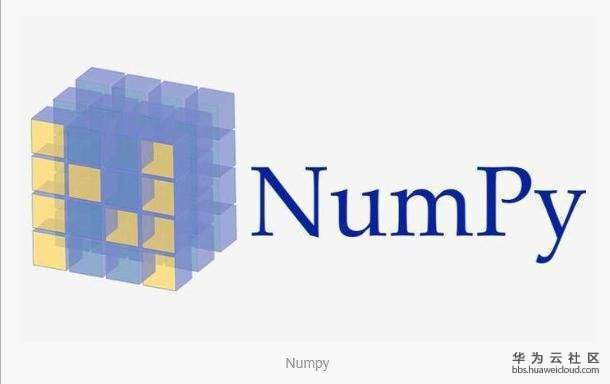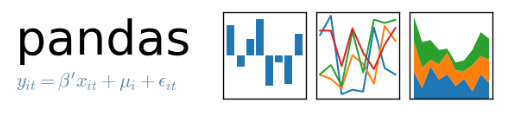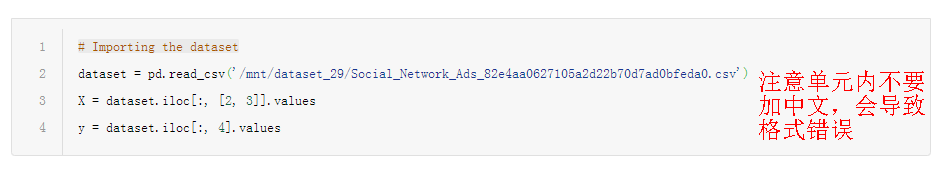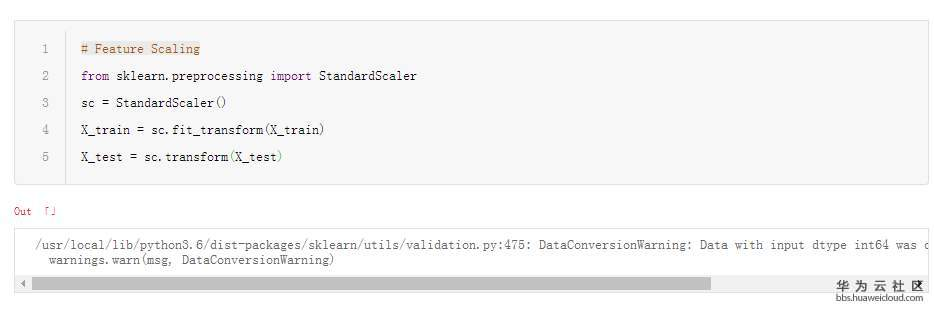[技术干货] 分类与预测——logistic回归分析

# 【Python算法】分类与预测——logistic回归分析

1.logistic回归定义

logistic回归是一种广义线性回归（generalized linear model），因此与多重线性回归分析有很多相同之处。它们的模型形式基本上相同，都具有 w‘x+b，其中w和b是待求参数，其区别在于他们的因变量不同，多重线性回归直接将w‘x+b作为因变量，即y =w‘x+b，而logistic回归则通过函数L将w‘x+b对应一个隐状态p，p =L(w‘x+b),然后根据p 与1-p的大小决定因变量的值。如果L是logistic函数，就是logistic回归，如果L是多项式函数就是多项式回归。logistic回归的因变量可以是二分类的，也可以是多分类的，但是二分类的更为常用，也更加容易解释，多类可以使用softmax方法进行处理。实际中最为常用的就是二分类的logistic回归。

2.操作系统

操作机：Linux_Ubuntu
操作机默认用户：root

3.实验工具Python是一种计算机程序设计语言。是一种动态的、面向对象的脚本语言，最初被设计用于编写自动化脚本(shell)，随着版本的不断更新和语言新功能的添加，越来越多被用于独立的、大型项目的开发。Python已经成为最受欢迎的程序设计语言之一。自从2004年以后，python的使用率呈线性增长。2011年1月，它被TIOBE编程语言排行榜评为2010年度语言。
由于Python语言的简洁性、易读性以及可扩展性，在国外用Python做科学计算的研究机构日益增多，一些知名大学已经采用Python来教授程序设计课程。例如卡耐基梅隆大学的编程基础、麻省理工学院的计算机科学及编程导论就使用Python语言讲授。

众多开源的科学计算软件包都提供了Python的调用接口，例如著名的计算机视觉库OpenCV、三维可视化库VTK、医学图像处理库ITK。而Python专用的科学计算扩展库就更多了，例如如下3个十分经典的科学计算扩展库：NumPy、SciPy和matplotlib，它们分别为Python提供了快速数组处理、数值运算以及绘图功能。因此Python语言及其众多的扩展库所构成的开发环境十分适合工程技术、科研人员处理实验数据、制作图表，甚至开发科学计算应用程序。

4.NumpyNumPy系统是Python的一种开源的数值计算扩展。这种工具可用来存储和处理大型矩阵，比Python自身的嵌套列表（nested list structure)结构要高效的多（该结构也可以用来表示矩阵（matrix））。
NumPy（Numeric Python）提供了许多高级的数值编程工具，如：矩阵数据类型、矢量处理，以及精密的运算库。专为进行严格的数字处理而产生。多为很多大型金融公司使用，以及核心的科学计算组织如：Lawrence Livermore，NASA用其处理一些本来使用C++，Fortran或Matlab等所做的任务。

5.scikit-learnscikit-learn，Python 中的机器学习，简单高效的数据挖掘和数据分析工具，可供大家使用，可在各种环境中重复使用，建立在 NumPy，SciPy 和 matplotlib 上开放源码，可商业使用 - BSD license。

6.MatplotlibMatplotlib 是一个 Python 的 2D绘**，它以各种硬拷贝格式和跨平台的交互式环境生成出版质量级别的图形。通过 Matplotlib，开发者可以仅需要几行代码，便可以生成绘图，直方图，功率谱，条形图，错误图，散点图等。

7.pandasPython Data Analysis Library 或 pandas 是基于NumPy 的一种工具，该工具是为了解决数据分析任务而创建的。Pandas 纳入了大量库和一些标准的数据模型，提供了高效地操作大型数据集所需的工具。pandas提供了大量能使我们快速便捷地处理数据的函数和方法。你很快就会发现，它是使Python成为强大而高效的数据分析环境的重要因素之一。

8.导入所需要的库:numpy,matplotlib.pyplot,pandas

```# Importing the libraries
import numpy as np
import matplotlib.pyplot as plt
import pandas as pd```

9.加载数据集

```# Importing the dataset
dataset = pd.read_csv('/mnt/dataset_29/Social_Network_Ads_82e4aa0627105a2d22b70d7ad0bfeda0.csv')   注意：数据集以实际位置为准
X = dataset.iloc[:, [2, 3]].values
y = dataset.iloc[:, 4].values```10.将数据集分割为训练集和测试集

```# Splitting the dataset into the Training set and Test set
from sklearn.model_selection import train_test_split
X_train, X_test, y_train, y_test = train_test_split(X, y, test_size = 0.25, random_state = 0)```

11.特征缩放

```# Feature Scaling
from sklearn.preprocessing import StandardScaler
sc = StandardScaler()
X_train = sc.fit_transform(X_train)
X_test = sc.transform(X_test)```

``

12.使用逻辑回归对数据进行处理

```from sklearn.linear_model import LogisticRegression
classifier = LogisticRegression()
classifier.fit(X_train, y_train)```13.对测试集进行分类

```# Predicting the Test set results
y_pred = classifier.predict(X_test)```

14.制造混淆矩阵评估分类器性能

```from sklearn.metrics import confusion_matrix
cm = confusion_matrix(y_test, y_pred)```

15.绘制训练集和测试集

```from matplotlib.colors import ListedColormap
X_set,y_set=X_train,y_train
X1,X2=np. meshgrid(np. arange(start=X_set[:,0].min()-1, stop=X_set[:, 0].max()+1, step=0.01),
np. arange(start=X_set[:,1].min()-1, stop=X_set[:,1].max()+1, step=0.01))
plt.contourf(X1, X2, classifier.predict(np.array([X1.ravel(),X2.ravel()]).T).reshape(X1.shape),
alpha = 0.75, cmap = ListedColormap(('red', 'green')))
plt.xlim(X1.min(),X1.max())
plt.ylim(X2.min(),X2.max())
for i,j in enumerate(np. unique(y_set)):
plt.scatter(X_set[y_set==j,0],X_set[y_set==j,1],
c = ListedColormap(('red', 'green'))(i), label=j)

plt. title(' LOGISTIC(Training set)')
plt. xlabel(' Age')
plt. ylabel(' Estimated Salary')
plt. legend()
plt. show()``````X_set,y_set=X_test,y_test
X1,X2=np. meshgrid(np. arange(start=X_set[:,0].min()-1, stop=X_set[:, 0].max()+1, step=0.01),
np. arange(start=X_set[:,1].min()-1, stop=X_set[:,1].max()+1, step=0.01))

plt.contourf(X1, X2, classifier.predict(np.array([X1.ravel(),X2.ravel()]).T).reshape(X1.shape),
alpha = 0.75, cmap = ListedColormap(('red', 'green')))
plt.xlim(X1.min(),X1.max())
plt.ylim(X2.min(),X2.max())
for i,j in enumerate(np. unique(y_set)):
plt.scatter(X_set[y_set==j,0],X_set[y_set==j,1],
c = ListedColormap(('red', 'green'))(i), label=j)

plt. title(' LOGISTIC(Test set)')
plt. xlabel(' Age')
plt. ylabel(' Estimated Salary')
plt. legend()
plt. show()```